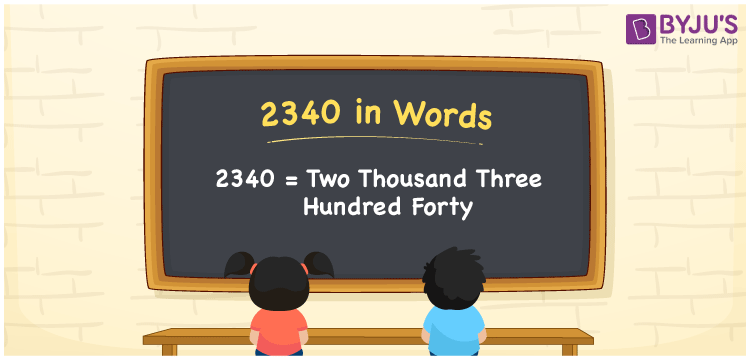# 2340 in words

2340 in words is written as Two Thousand Three Hundred and Forty. 2340 represents the count or value. The article on Counting Numbers can give you an idea about count or counting. The number 2340 is used in expressions that relate to money, distance, length, year and others. Let us consider an example for 2340. ”A TV daily soap opera has completed Two Thousand Three Hundred and Forty episodes”.

 2340 in words Two Thousand Three Hundred and Forty Two Thousand Three Hundred and Forty in Numbers 2340

## 2340 in English Words## How to Write 2340 in Words?

We can convert 2340 to words using a place value chart. The number 2340 has 4 digits, so let’s make a chart that shows the place value up to 4 digits.

 Thousands Hundreds Tens Ones 2 3 4 0

Thus, we can write the expanded form as:

2 × Thousand + 3 × Hundred + 4 × Ten + 0 × One

= 2 × 1000 + 3 × 100 + 4 × 10 + 0 × 1

= 2340

= Two Thousand Three Hundred and Forty.

2340 is the natural number that is succeeded by 2339 and preceded by 2341.

2340 in words – Two Thousand Three Hundred and Forty.

Is 2340 an odd number? – No.

Is 2340 an even number? – Yes.

Is 2340 a perfect square number? – No.

Is 2340 a perfect cube number? – No.

Is 2340 a prime number? – No.

Is 2340 a composite number? – Yes.

## Solved Example

1. Write the number 2340 in expanded form

Solution: 2 × 1000 + 3 × 100 + 4 × 10 + 0 × 1

We can write 2340 = 2000 + 300 + 40 + 0

= 2 × 1000 + 3 × 100 + 4 × 10 + 0 × 1

## Frequently Asked Questions on 2340 in words

Q1

### How to write 2340 in words?

2340 in words is written as Two Thousand Three Hundred and Forty.
Q2

### Is 2340 a perfect square number?

No. 2340 is not a perfect square number.
Q3

### Is 2340 a prime number?

No. 2340 is not a prime number.